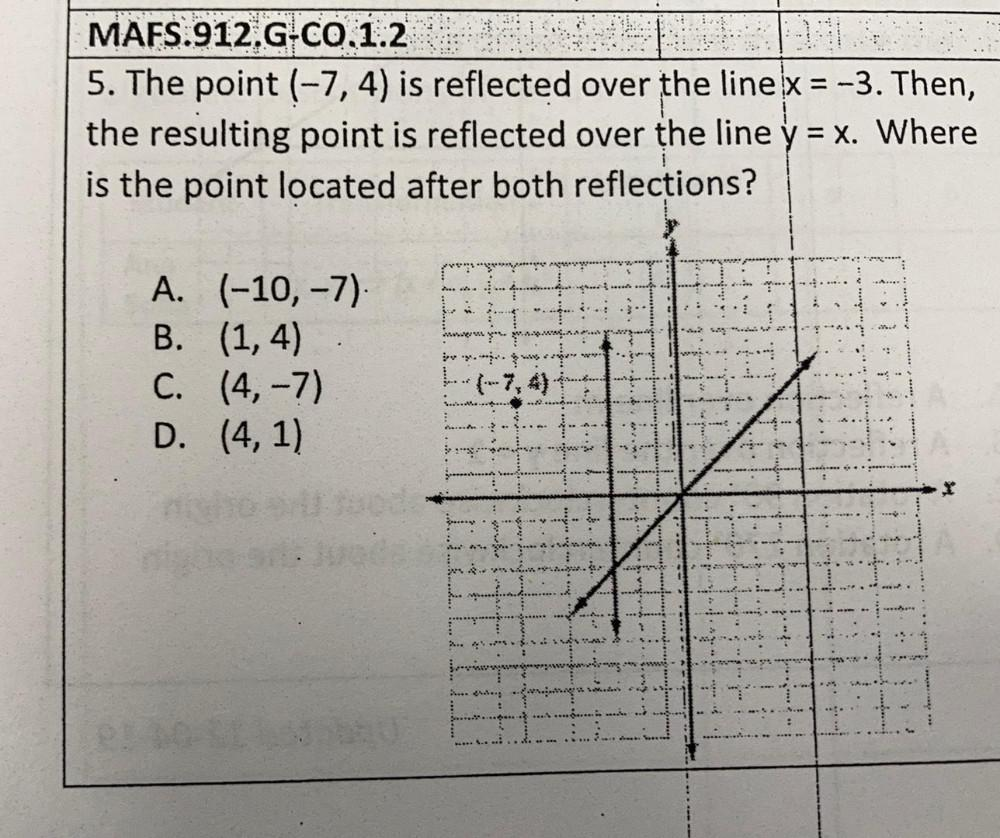Question:

# = MAFS:912.G.CO,1.2 5. The point (-7,4) is reflected over the line x = -3. Then, the resulting point is reflected over the line= MAFS:912.G.CO,1.2 5. The point (-7,4) is reflected over the line x = -3. Then, the resulting point is reflected over the line y = x. Where is the point located after both reflections? A. (-10,-7). B. (1,4) C. (4, -7) D. (4,1) py. 2 ... A A.